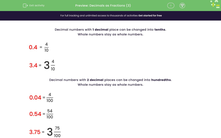# Decimals as Fractions (3)

In this worksheet, students must read and write decimal numbers up to thousandths as fractions or mixed numbers.Key stage:  KS 2

Curriculum topic:   Number: Fractions, Decimals and Percentages

Curriculum subtopic:   Know Decimal Numbers

Difficulty level:#### Worksheet Overview

Decimal numbers with 1 decimal place can be changed into tenths.

Whole numbers stay as whole numbers.

 0.4 = 4 10

 3.4 = 3 4 10

Decimal numbers with 2 decimal places can be changed into hundredths.

Whole numbers stay as whole numbers.

 0.04 = 4 100

 0.54 = 54 100

 3.75 = 3 75 100

Decimal numbers with 3 decimal places can be changed into thousandths.

Whole numbers stay as whole numbers.

 0.004 = 4 1000

 0.054 = 54 1000

 3.715 = 3 715 1000

The reverse is also true.

Example 1

Write the following fraction as a decimal.

 5 1000

5 thousandths is 0.005 with 3 decimal places.

(Use zeros to fill in the spaces.)

Example 2

Write the following mixed number as a decimal.

 4 15 1000

15 thousandths is 0.015 with 3 decimal places.

The 4 is a whole number and stays the same.